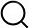# Also in the Article

Statistical analysis

Procedure

Data are presented as means ± SD, unless otherwise noted. All statistical analysis was performed in Python 3.6, MATLAB 9.3, or GraphPad Prism 7.0d. For comparisons of means in samples with normal distributions and homogeneous variances (as indicated by a Levene’s test), ANOVA was used for comparisons between two or more means, followed by Bonferroni P value correction for multiple comparisons. In cases of a non-normal distribution (as indicated by a Shapiro Wilk test) or unequal variances (Levene’s test), a nonparametric Kruskal-Wallis test was used for comparisons between two or more means, followed by Bonferroni P value correction for multiple comparisons. Linear least squares regression was used for calculation of correlations between group averages of EEG band powers, cardiovascular measures, and tracer MPIs. Significance was ascribed at P < 0.05.

Q&A